# Absolute Stability for Quantized System

This example shows how to enforce absolute stability when a linear time-invariant system is in feedback interconnection with a static nonlinearity that belongs to a conic sector.

### Feedback Connection

Consider the feedback connection as shown in Figure 1.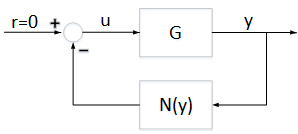Figure 1: Feedback connection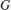is a linear time invariant system, and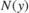is a static nonlinearity that belongs to a conic sector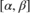(where); that is,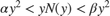For this example,is the following discrete-time system.

A = [0.9995, 0.0100, 0.0001; -0.0020, 0.9995, 0.0106; 0, 0, 0.9978]; B = [0, 0.002, 0.04]'; C = [2.3948, 0.3303, 2.2726]; D = 0; G = ss(A,B,C,D,0.01); 

### Sector Bounded Nonlinearity

In this example, the nonlinearityis the logarithmic quantizer, which is defined as follows: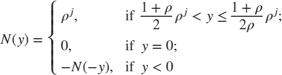where,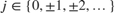. This quantizer belongs to a sector bound. For example, if, then the quantizer belongs to the conic sector [0.1818,1.8182].

% Quantizer parameter rho = 0.1; % Lower bound alpha = 2*rho/(1+rho) % Upper bound beta = 2/(1+rho) 
alpha = 0.1818 beta = 1.8182 

Plot the sector bounds for the quantizer.

PlotSectorBound(rho)represents the quantization density, where. Ifis larger, then the quantized value is more accurate. For more details about this quantizer, see .

### Conic Sector Condition for Absolute Stability

The conic sector matrix for the quantizer is given by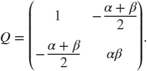To guarantee stability of the feedback connection in Figure 1, the linear systemneeds to satisfywhere,and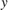are the input and output of, respectively.

This condition can be verified by checking if the sector index,, is less than 1.

Define the conic sector matrix for a quantizer with.

Q = [1,-(alpha+beta)/2;-(alpha+beta)/2,alpha*beta]; 

Get the sector index for Q and G.

R = getSectorIndex([1;-G],-Q) 
R = 1.8247 

Since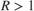, the closed-loop system is not stable. To see this instability, use the following Simulink model.

mdl = 'DTQuantization'; open_system(mdl)sim(mdl) open_system('DTQuantization/output')From the output trajectory, it can be seen that the closed-loop system is not stable. This is because the quantizer withis too coarse.

Increase the quantization density by letting. The quantizer belongs to the conic sector [0.4,1.6].

% Quantizer parameter rho = 0.25; % Lower bound alpha = 2*rho/(1+rho) % Upper bound beta = 2/(1+rho) 
alpha = 0.4000 beta = 1.6000 

Plot the sector bounds for the quantizer.

PlotSectorBound(rho)Define the conic sector matrix for a quantizer with.

Q = [1,-(alpha+beta)/2;-(alpha+beta)/2,alpha*beta]; 

Get the sector index for Q and G.

R = getSectorIndex([1;-G],-Q) 
R = 0.9702 

The quantizer withsatisfies the conic sector condition for stability of the feedback connection since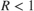.

Run the Simulink model with.

sim(mdl) open_system('DTQuantization/output')As indicated by the sector index, the closed-loop system is stable.

### Reference

 M. Fu and L. Xie,"The sector bound approach to quantized feedback control," IEEE Transactions on Automatic Control 50(11), 2005, 1698-1711.